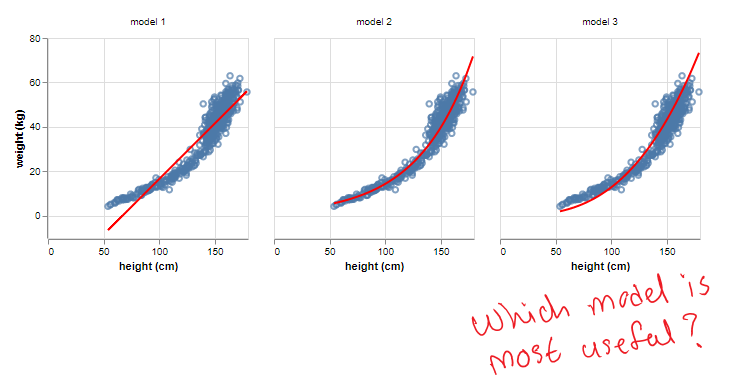# Bayesian Inference: Beyond Estimating Statistical ModelsBayesian Inference: Beyond Estimating Statistical Models. This article will show how to incorporate Bayesian inference to build scientific models and the benefits of doing so.

## Introduction

Let’s divide the universe of models into two types: statistical and scientific. Both classes of models aims to understand the relationship between a target variable i.e. the y, and a set of features i.e. the *x. *The former aims to find statistically sound relationships based on data while the latter has the property of being able to describe a cause and effect relationship.

All the examples in this series have used statistical models e.g. GLMs to illustrate a concept in Bayesian inference. However, Bayesian inference is just as relevant to building scientific models.

This article will show how to incorporate Bayesian inference to build scientific models and the benefits of doing so.

The code to reproduce the results described in this article can be found in this notebook.

## Problem

To keep the math simple, let’s imagine we want to build a model to predict a person’s weight given height.

## Most popular Data Science and Machine Learning courses — July 2020

Most popular Data Science and Machine Learning courses — August 2020. This list was last updated in August 2020 — and will be updated regularly so as to keep it relevant

## Statistics for Data Science

Statistics for Data Science and Machine Learning Engineer. I’ll try to teach you just enough to be dangerous, and pique your interest just enough that you’ll go off and learn more.

## Difference between Machine Learning, Data Science, AI, Deep Learning, and Statistics

In this article, I clarify the various roles of the data scientist, and how data science compares and overlaps with related fields such as machine learning, deep learning, AI, statistics, IoT, operations research, and applied mathematics.

## 15 Machine Learning and Data Science Project Ideas with Datasets

Learning is a new fun in the field of Machine Learning and Data Science. In this article, we’ll be discussing 15 machine learning and data science projects.

## The Role of Bayesian Statistics In A Post-COVID World

COVID-19 showed us that forecasts based on past data can become outdated very quickly. One of the central concepts in Bayesian statistics is that of a posterior distribution.